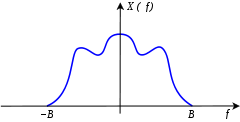# Nyquist rate

﻿
Nyquist rateSpectrum of a bandlimited signal as a function of frequency

In signal processing, the Nyquist rate, named after Harry Nyquist, is two times the bandwidth of a bandlimited signal or a bandlimited channel. This term is used to mean two different things under two different circumstances:

1. as a lower bound for the sample rate for alias-free signal sampling (not to be confused with the Nyquist frequency, which is half the sampling rate of a discrete-time system) and
2. as an upper bound for the symbol rate across a bandwidth-limited baseband channel such as a telegraph line or passband channel such as a limited radio frequency band or a frequency division multiplex channel.

## Nyquist rate relative to sampling

The Nyquist rate is the minimum sampling rate required to avoid aliasing, equal to twice the highest frequency contained within the signal.$f_N \ \stackrel{\mathrm{def}}{=}\ 2 B\,$

where$B\,$ is the highest frequency at which the signal can have nonzero energy.

To avoid aliasing, the sampling rate must exceed the Nyquist rate:$f_S > f_N.\,$

A signal whose positive-frequency range of significant energy is (0, B), as depicted above, is called baseband or lowpass. But when the frequency range is (AB), for some A > B − A, it is called bandpass. In that case, aliasing is not necessarily detrimental, and sampling below the Nyquist rate, called bandpass sampling, is sometimes done. With careful design, a rate as low as 2(B − A) may be achievable, and it is equivalent to mixing (heterodyne) the signal into the frequency range (0, B − A), whose Nyquist rate is 2(B − A). An even lower Nyquist rate can be achieved for a bandpass signal, such as amplitude modulation, whose energy distribution in (AB) is symmetrical. In that case, the homodyne mixer translates the signal frequencies by (A + B)/2, with a synchronized phase, which moves the highest component to (B − A)/2 and the Nyquist rate to just (B − A).

## Nyquist rate relative to signaling

Long before Harry Nyquist had his name associated with sampling, the term Nyquist rate was used differently, with a meaning closer to what Nyquist actually studied. Quoting Harold S. Black's 1953 book Modulation Theory, in the section Nyquist Interval of the opening chapter Historical Background:

"If the essential frequency range is limited to B cycles per second, 2B was given by Nyquist as the maximum number of code elements per second that could be unambiguously resolved, assuming the peak interference is less half a quantum step. This rate is generally referred to as signaling at the Nyquist rate and 1/(2B) has been termed a Nyquist interval." (bold added for emphasis; italics as in the original)

According to the OED, this may be the origin of the term Nyquist rate.

Nyquist's famous 1928 paper was a study on how many pulses (code elements) could be transmitted per second, and recovered, through a channel of limited bandwidth. Signaling at the Nyquist rate meant putting as many code pulses through a telegraph channel as its bandwidth would allow. Shannon used Nyquist's approach when he proved the sampling theorem in 1948, but Nyquist did not work on sampling per se.

Black's later chapter on "The Sampling Principle" does give Nyquist some of the credit for some relevant math:

"Nyquist (1928) pointed out that, if the function is substantially limited to the time interval T, 2BT values are sufficient to specify the function, basing his conclusions on a Fourier series representation of the function over the time interval T."

Wikimedia Foundation. 2010.

### Look at other dictionaries:

• Nyquist rate — noun (telecommunication) the lowest sampling rate that will permit accurate reconstruction of a sampled analog signal • Topics: ↑telecommunication, ↑telecom • Hypernyms: ↑sampling rate …   Useful english dictionary

• Nyquist — is a surname of Swedish and Norwegian origin which may refer to: People Arild Nyquist (1937–2004), Norwegian novelist, lyricist, writer and musician Harry Nyquist (1889–1976), Swedish American engineer Kari Nyquist (born 1918), Norwegian ceramist …   Wikipedia

• Nyquist–Shannon sampling theorem — Fig.1: Hypothetical spectrum of a bandlimited signal as a function of frequency The Nyquist–Shannon sampling theorem, after Harry Nyquist and Claude Shannon, is a fundamental result in the field of information theory, in particular… …   Wikipedia

• Nyquist frequency — Not to be confused with Nyquist rate. Frequencies above ƒs/2 (the Nyquist frequency) have an alias below ƒs/2, whose value is given by this graph. ƒs/2 is also called folding frequency, because of the symmetry between 0 and ƒs. The Nyquist… …   Wikipedia

• Nyquist ISI criterion — Raised cosine response meets the Nyquist ISI criterion. Consecutive raised cosine impulses demonstrate the zero ISI property between transmitted symbols at the sampling instants. At t=0 the middle pulse is at its maximum and the sum of other… …   Wikipedia

• Nyquist-Formel — Wärmerauschen, thermisches Rauschen, Widerstandsrauschen, Nyquist Rauschen, Johnson Rauschen oder Johnson Nyquist Rauschen genannt, ist ein weitgehend weißes Rauschen, das aus der thermischen Bewegung der Ladungsträger in elektrischen… …   Deutsch Wikipedia

• Nyquist-Rauschen — Wärmerauschen, thermisches Rauschen, Widerstandsrauschen, Nyquist Rauschen, Johnson Rauschen oder Johnson Nyquist Rauschen genannt, ist ein weitgehend weißes Rauschen, das aus der thermischen Bewegung der Ladungsträger in elektrischen… …   Deutsch Wikipedia

• Nyquist, Harry — ▪ American physicist born Feb. 7, 1889, Nilsby, Sweden died April 4, 1976, Harlingen, Texas, U.S.       American physicist and electrical and communications engineer, a prolific inventor who made fundamental theoretical and practical… …   Universalium

• Harry Nyquist — (1889 1976) Born February 7, 1889( …   Wikipedia

• Bit rate — Bit rates Decimal prefixes (SI) Name Symbol Multiple kilobit per second kbit/s 103 megabit per second Mbit/s 106 gigabit per second Gbit/s 109 …   Wikipedia